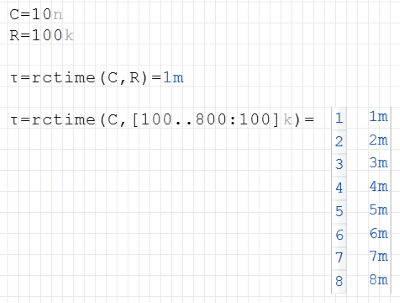# RCTime Function

Calculates the time constant τ (Tau) vof a resistor and a capacitor

## Calculate time constant

The RCTime function calculates the time constant τ (tau) of an RC circuid (low pass). Real numbers or lists can be passed as parameters.

The function is defined as $$\displaystyle τ=R · C$$

### Syntax

RCTime (R, C)

RCTime (R, List C)

RCTime (List R, C)

RCTime (List R, List C)

### Example